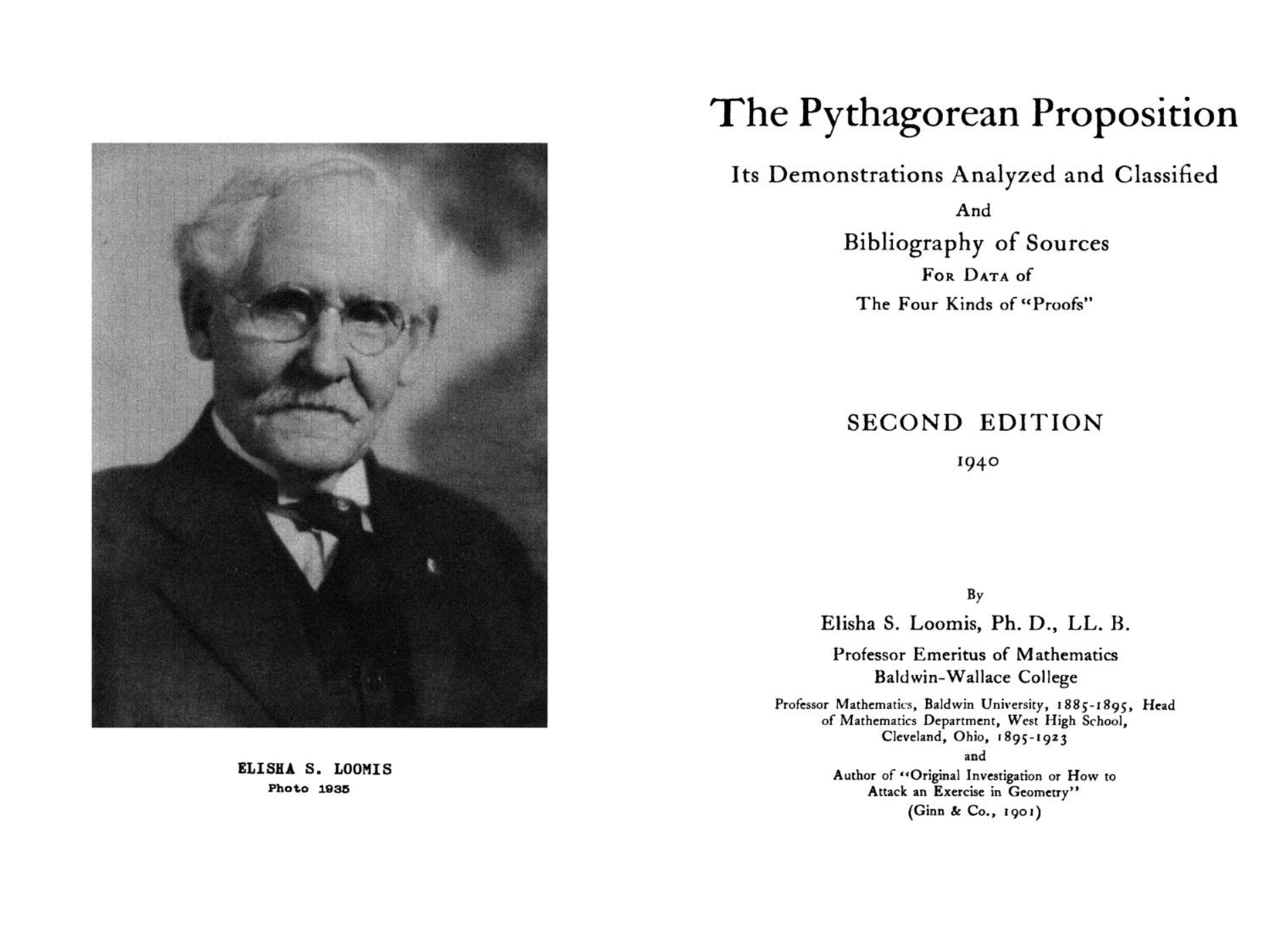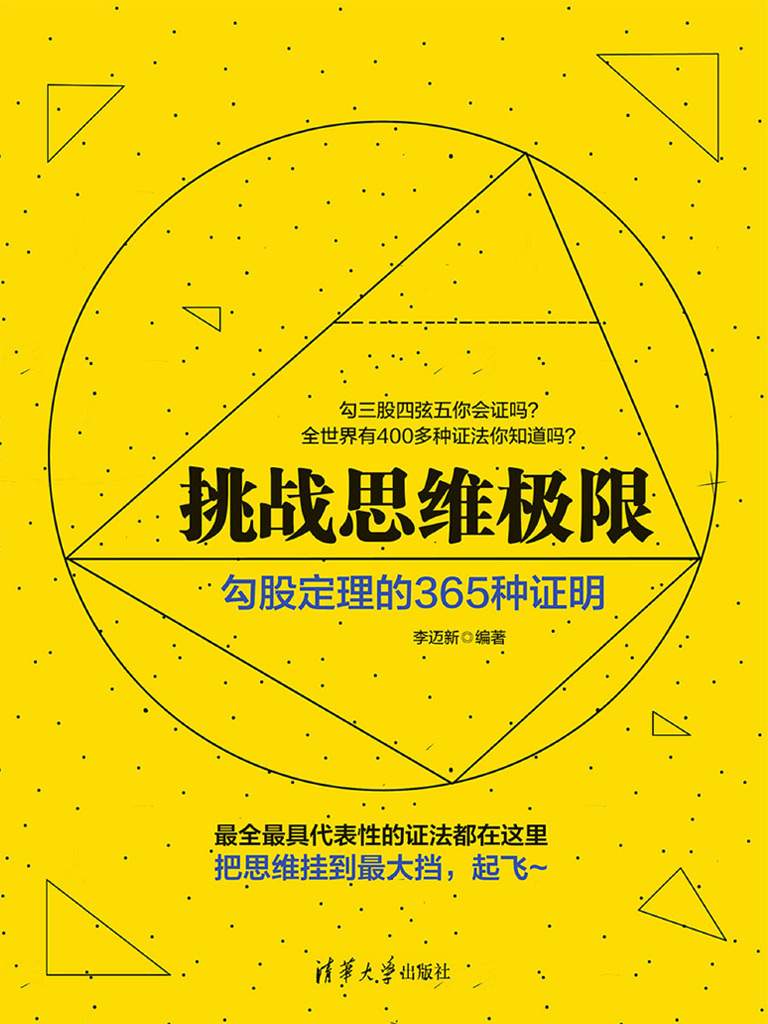# 勾股定理及其证明

## 勾股定理## 欧几里得证法$$S_{ABDW}=2S_{\triangle AWC}=2S_{\triangle ABP}=S_{APYX}$$

## 赵爽：勾股圆方图$$\begin{array}{l} \because \quad (b-a)^2+\dfrac{1}{2}ab\times 4=c^2 \\ \\ \therefore \quad a^2+b^2=c^2 \end{array}$$

## 刘徽：青出朱入图## 达芬奇证法$$\begin{array}{l} \because \quad a^2+b^2+\dfrac{1}{2}ab\times 2=c^2+ \dfrac{1}{2}ab\times 2 \\ \\ \therefore \quad a^2+b^2=c^2 \end{array}$$

## 总统法$$\begin{array}{l} \because \quad \dfrac{1}{2}(a+b)\times (a+b)=\dfrac{1}{2}c^2+\dfrac{1}{2}ab\times 2 \\ \\ \therefore \quad (a+b)^2=c^2+2ab \\ \\ \therefore \quad a^2+2ab+b^2=c^2+2ab \\ \\ \therefore \quad a^2+b^2=c^2 \end{array}$$## 勾股定理与高考题：“罪大恶极”的数学家——潘承彪（作者：萨苏）